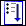> > Calculate a clash analysis

Calculate a clash analysis
You can run (or calculate) a saved analysis at any time. When you calculate an analysis, the issue browser window opens.
To calculate a saved analysis,
1. Click Analysis and then, in the Verify group, clickCalculate.
2. Double-click an analysis in the Structure Browser.
OR
1. Right-click an analysis in the Structure Browser.
2. Click Calculate.